GeeksforGeeks App
Open AppBrowser
Continue

## Related Articles

• NCERT Solutions for Class 8 Maths

# Class 8 NCERT Solutions – Chapter 11 Mensuration – Exercise 11.1

### Question 1. A square and a rectangular field with measurements as given in the figure have the same perimeter. Which field has a larger area?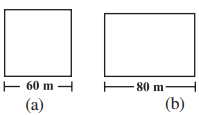Solution:

Given,

Side of a square = 60 m

Length, l = 80 m

According to question,

Perimeter of rectangular field = Perimeter of square field

According to formula,

2(l + b) = 4 × Side

2(80 + b) = 4 × 60

160 + 2b = 240

b = 40

Therefore, Breadth of the rectangle is 40 m.

Now, Area of Square field = (side)2 = (60)2 = 3600 m2

And Area of Rectangular field = length × breadth = 80 × 40 = 3200 m2

Hence, area of square field is larger.

### Question 2. Mrs.Kaushik has a square plot with the measurement as shown in the figure. She wants to construct a house in the middle of the plot. A garden is developed around the house. Find the total cost of developing a garden around the house at the rate of Rs. 55 per m2.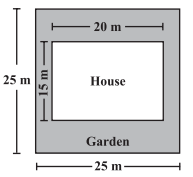Solution:

Given,

Side of a square = 25 m

According to formula,

Area of square plot =  square of a side = (side)2 = (25)2 = 625

Therefore, the area of a square plot is 625 m2

Length of the house = 20 m and

Breadth of the house = 15 m

Area of the house = length × breadth = 20 × 15 = 300 m2

Area of garden = Area of square plot – Area of house = 625 – 300 = 325 m2

Therefore, per unit cost of making garden is Rs. 55

Total Cost of developing the garden of 325 sq. m = Rs. 55 × 325 = Rs. 17,875

### Question 3. The shape of a garden is rectangular in the middle and semi-circular at the ends as shown in the diagram. Find the area and the perimeter of this garden [Length of rectangle is 20 – (3.5 + 3.5 meters)]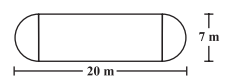Solution:

Given,

Total length = 20 m

Diameter of semi circle = 7 m

Therefore, Radius of semi circle = 7/2 = 3.5 m

Length of rectangular field = 20 – (3.5 + 3.5) = 20 – 7 = 13 m

Breadth of the rectangular field = 7 m

Area of rectangular field = l × b = 13 × 7= 91m2

Area of two semi circles = 2 × (1/2) × pi × r2

= 2 × (1/2) × 22/7 × 3.5 × 3.5

= 38.5 m2

Area of garden = 91 + 38.5 = 129.5 m2

Now Perimeter of two semi circles =  2.pi.r = 2 × (22/7) × 3.5  = 22 m

Therefore, Perimeter of garden = 22 + 13 + 13 = 48 m

### Question 4. A flooring tile has the shape of a parallelogram whose base is 24 cm and the corresponding height is 10 cm. How many such tiles are required to cover a floor of area 1080 m2? [If required you can split the tiles in whatever way you want to fill up the corners]

Solution:

Given,

Base of flooring tile = 24 cm = 0.24 m

Corresponding height of a flooring tile = 10 cm = 0.10 m

Now Area of flooring tile = Base × Altitude = 0.24 × 0.10 = 0.024

Area of flooring tile is 0.024m2

Number of tiles required to cover the floor = Area of floor/Area of one tile = 1080/0.024 = 45000 tiles

Hence 45000 tiles are required to cover the floor.

### Question 5. An ant is moving around a few food pieces of different shapes scattered on the floor. For which food-piece would the ant have to take a longer round? Remember, the circumference of a circle can be obtained by using the expression C = 2.pi.r,wherer is the radius of the circle.

Solution:

(a)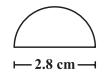Radius =  Diameter/2 = 2.8/2 cm = 1.4 cm

Circumference of semi-circle = pi.r = (22/7)×1.4 = 4.4

Circumference of semi-circle is 4.4 cm

Total distance covered by the ant = Circumference of semi – circle + Diameter = 4.4 + 2.8 = 7.2 cm

(b)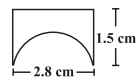Diameter of semi-circle = 2.8 cm

Radius =  Diameter/2 = 2.8/2  = 1.4 cm

Circumference of semi-circle = r = (22/7) × 1.4 = 4.4 cm

Total distance covered by the ant= 1.5 + 2.8 + 1.5 + 4.4 = 10.2 cm

(c)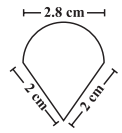Diameter of semi-circle = 2.8 cm

Radius =  Diameter/2 = 2.8/2 = 1.4 cm

Circumference of semi-circle =  pi.r =  (22/7) × 1.4 = 4.4 cm

Total distance covered by the ant = 2 + 2 + 4.4 = 8.4 cm

After analyzing results of three figures, we concluded that for figure (b) food piece, the ant would take a longer round.

My Personal Notes arrow_drop_up
Related Tutorials